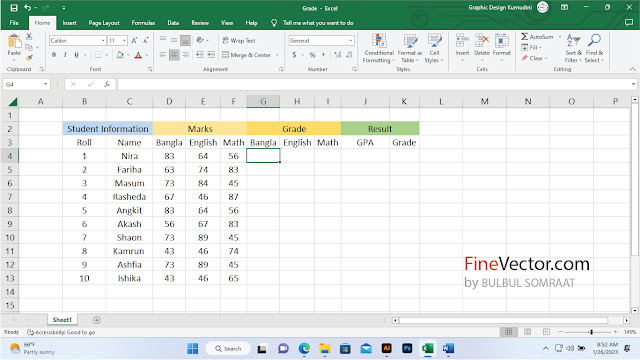## How to create Result sheet in Microsoft ExcelGPA and Grade in Microsoft Excel.

### Using Excel Formula:

1. First, we input data in Microsoft Excel like the example in the picture below. with all subjects that I want to make result sheet. then we calculate grade.2. We select the cell Bangla for calculating its grade point for each subject by using formula.
here we use marks for generating grade point. then we apply the formula.
=if(D4>=80,5,if(D4>=70,4,if(D4>=60,3.5,if(D4>=50,3,if(D4>=40,2,if(D4>=33,1,if(D4<33,0)))))))

3. here we found the grade point for each subject for each candidate.

4. Then we drug cursor for every subject to calculate grade point.

5. Now We calculate Grade Point Average (GPA).

6. Using formula for calculate GPA.

=IF(AND(G4>0,H4>0,I4>0),AVERAGE(G4:I4),0)

7. Here is the GPA calculated

9. Drug cursor for all candidate.

1.